# The physics (and mathematics) of gravitational microlensing

Gravitational microlensing is understood as the transient brightening of an observed star
resulting from the gravitational bending of its light by an intervening foreground 'lens' star.

The underlying physics is remarkably simple.

The deflection

• increases with the mass of the lens star (proportionally), and
• decreases with the separation of the light ray from the lens star (inversely proportionally).

The gravitational bending of light thereby differs significantly from the effect caused by an optical convex lens, but is rather similar to the deflection by a glass body in the shape of the foot of a wine glass.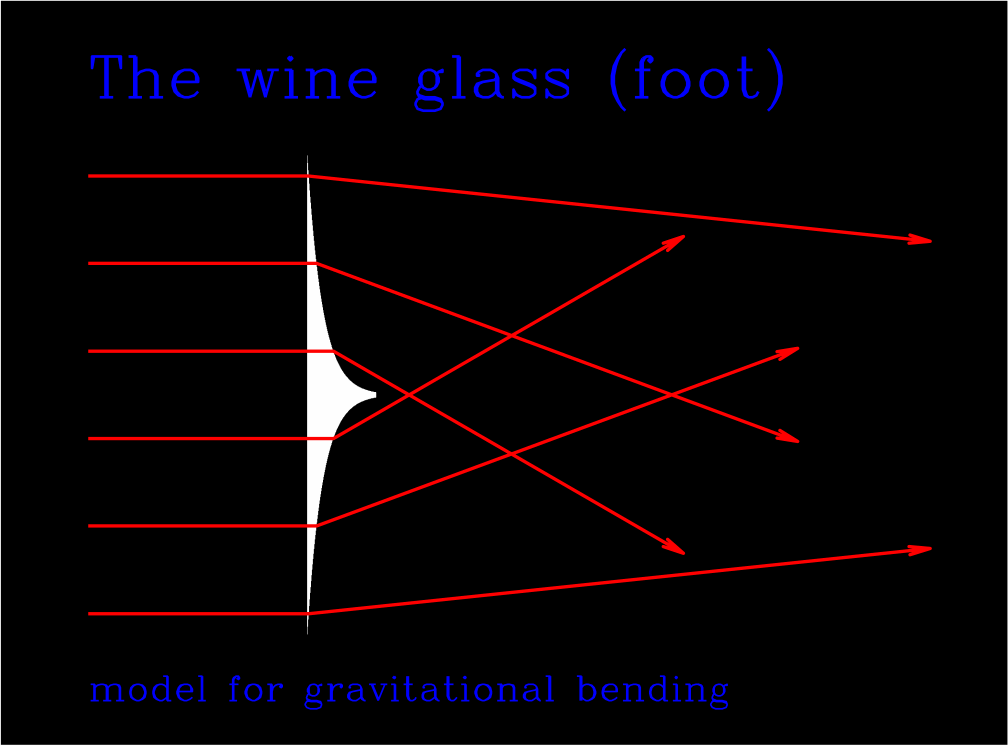Deflection of parallel light rays by a convex lens and by the foot of the wine glass, which represents an analogy to the gravitational bending of light. With the deflection decreasing towards its centre, the convex lens leads to a focussing. A different behaviour results for the light bending by massive bodies, where the deflection angle increases the closer the light ray approaches.

Explicitly, for a lens of mass M, the deflection law for a light ray at an impact separation &xi readswhere G denotes the universal gravitational constant, and c is the vacuum speed of light. This amount, as predicted by Einstein's theory of General Relativity, is in fact exactly twice the Newtonian value. This factor of two is however the only relevant contribution of General Relativity to the physics of gravitational microlensing.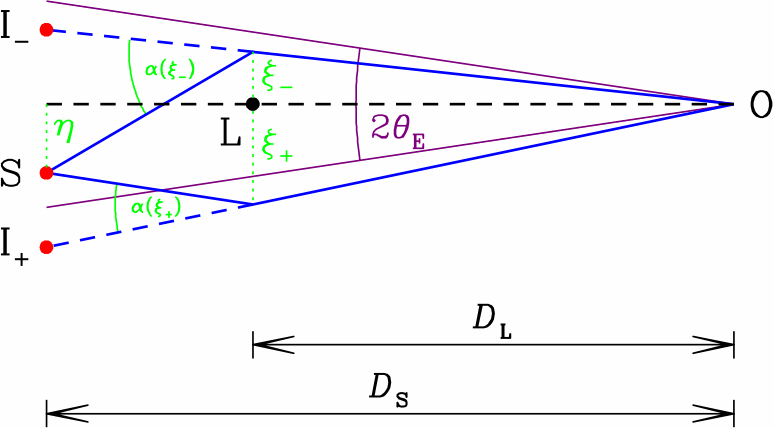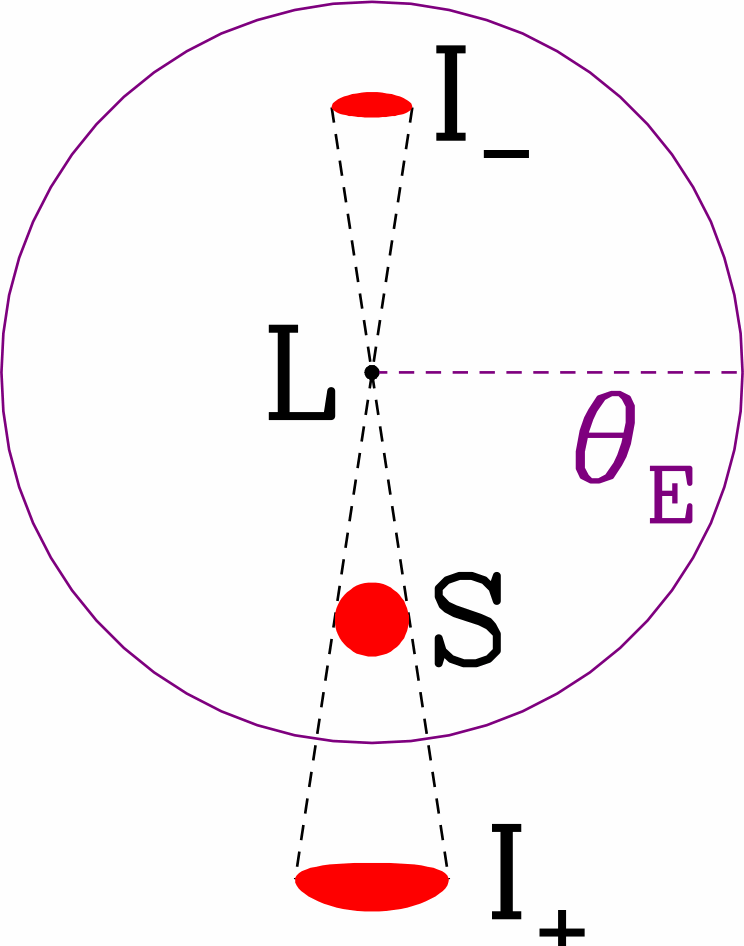Side view (left) and observer's view (right) on a source star (S) affected by gravitational microlensing due to a foreground lens star (L), where two possible light paths to the observer (O) correspond to two apparent images I- and I+.

As found by Einstein as early as 1912, geometry demands a ray-tracing equation resulting from the condition that light rays originating from the source star hit the observer. It provides the separation η of the light ray from the optical axis connecting lens star and observer as the light leaves the source at distance DS as a function of ξ, the separation of the light ray from the lens star at distance DL as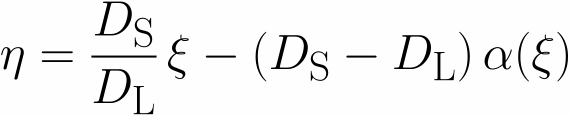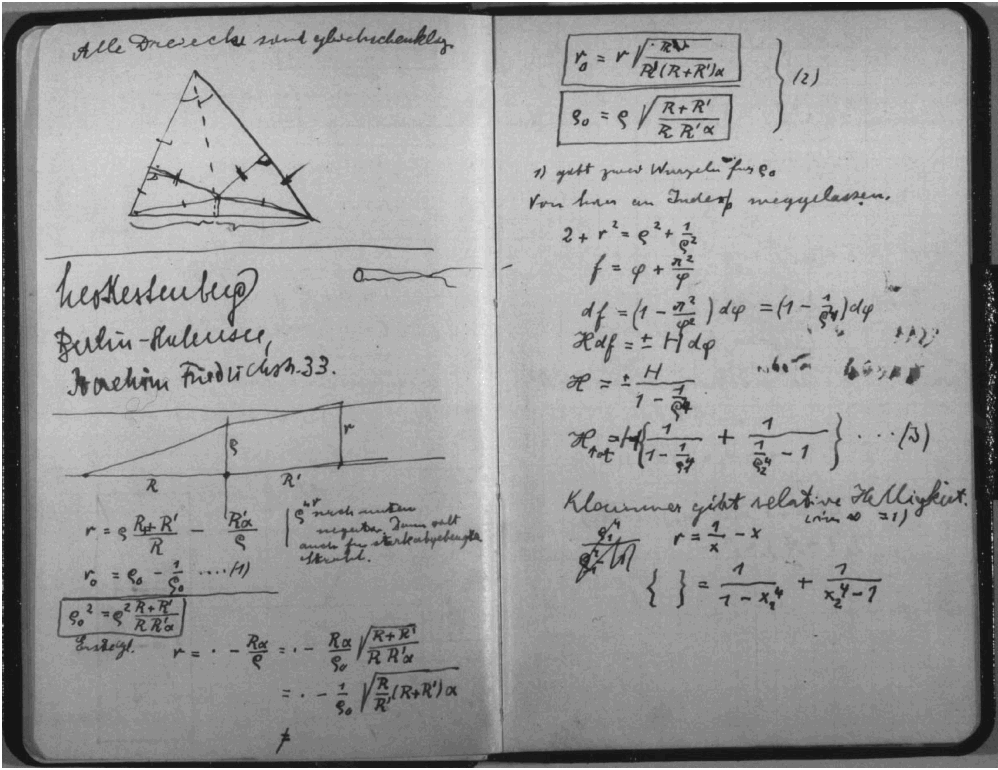First two pages of the discussion of gravitational microlensing in Einstein's notebook of 1912. © The Einstein Archives

Einstein also discovered that there is a unique characteristic scale, now called the angular Einstein radius θE, that absorbs all the physical parameters, namely the mass M of the lens star, as well as the lens and source distances DL and DS: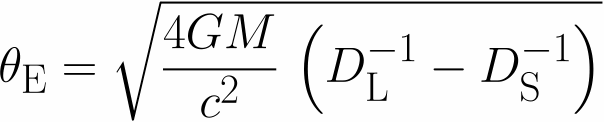With dimensionless angular coordinatesthe ray-tracing equation takes the simple formThis leads to a quadratic equation for the image positions x as function of the true source coordinate y, whose two solutions read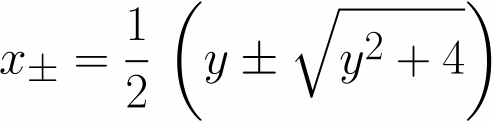It can easily be verified that one of the two images always falls inside the angular Einstein radius (|x| < 1), while the other one is always to be found outside (|x+| > 1), unless lens and source star are perfectly aligned (y = 0), in which case a ring-like image (Einstein ring) results, whose angular radius is equal to θE (given that x = 1).

Apart from the images appearing at angular positions different from that of the source star, gravitational lensing also accounts for a distortion, where the images are stretched radially by dx/dy and tangentially by x/y (given that the polar angle is conserved). Since this changes the solid angle from which light of the source star is received at the observer, a combined magnification A(y) of both images, given by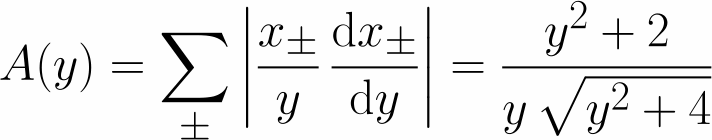is being observed, whereas the images themselves cannot be resolved by current optical telescopes, given that their separation is less than a milli-arcsecond.

While the source star always happens to be magnified (A > 1), and never de-magnified, the magnification is the larger the more closely source and lens star are aligned. Therefore, if stars can be assumed to move uniformly, microlensing light curves are symmetric with respect to a peak, which is reached at the epoch that marks the minimal angular impact between lens and source star.

Martin Dominik, 25 May 2008